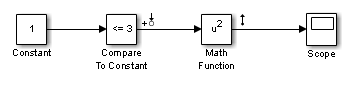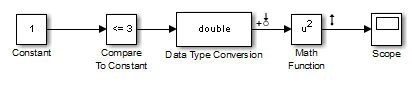## Linearize Blocks with Non-Floating-Point Signals or States

You can linearize blocks that have non-floating-point signals or states and have no preprogrammed exact linearization. Without additional configuration, such blocks automatically linearize to zero. For example, logical operator blocks have Boolean outputs and linearize to 0.

Linearizing blocks that have non-floating-point signals requires converting all signals to either double-precision or single-precision. This approach only works when your model can run correctly in full double or single precision.

When you have only a few blocks impacted by the non-floating-point data types, you can use a Data Type Conversion block to fix this issue.

When you have many nondouble precision signals, you can override all data types with double precision using the Fixed Point Tool.

### Override Data Type Using Data Type Conversion Block

Convert individual signals to double precision before linearizing the model by inserting a Data Type Conversion block. This approach works well for model that have only a few affected blocks.

After linearizing the model, remove the Data Type Conversion block from your model.

Note

Overriding non-floating-point data types is not appropriate when the model relies on these data types, such as relying on integer data types to perform truncation from floating-point values.

For example, consider the model configured to linearize the Square block at an operating point where the input is 1. The resulting linearized model should be 2, but the input to the Square block is Boolean. This signal with a non-floating-point data type results in a linearization of zero.In this case, inserting a Data Type Conversion block converts the input signal to the Square block to double precision. Doing so### Overriding Data Types Using Fixed Point Tool

When you linearize a model that contains non-floating-point data types but still runs correctly in full double or single precision, you can override all data types to be either double-precision or single-precision using the Fixed Point Tool. Use this approach when you have many non-floating-point precision signals.

After linearizing the model, restore your original settings.

Note

Overriding non-floating-point data types is not appropriate when the model relies on these data types, such as relying on integer data types to perform truncation from floats.

1. Open the Fixed Point Tool. In the Simulink® model window, on the Apps tab, in the Apps gallery, under Code Generation, click Fixed Point Tool.

2. In the Data type override menu, select either Double or Single

3. Restore settings when linearization is complete.